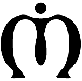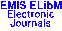Publications de l'Institut Mathématique, Nouvelle Série Vol. 82(96), pp. 155–163 (2007)

Previous Article

Contents of this Issue

Other Issues

ELibM Journals

ELibM Home

EMIS Home

Pick a mirror

## FIN-SET: A SYNTACTICAL DEFINITION OF FINITE SETS

### Slavisa B. Presic

Abstract: We state Fin-set, by which one founds the notion of finite sets in a syntactical way. Any finite set $\{a_1,a_2,\dots,a_n\}$ is defined as a well formed term of the form $S(a_1+(a_2+(\cdots+(a_{n-1}+a_n)\cdots)))$, where $+$ is a binary and $S$ a unary operational symbol. Related to the operational symbol $+$ the term-substitutions (1) are supposed. Definition of finite sets is called syntactical because by two algorithms Set-alg and Calc (below) one can effectively establish whether any given set-terms are equal or not equal.

All other notions of finite sets, like $\in$, ordered pair, Cartesian product, relation, function, cardinal number are defined as a corresponding term. Each of these definitions is recursive. For instance, $\in$ is defined by \begin{align*} &x\in S(a_1)\quad\text{iff}\quad x=a_1